## Wednesday, April 26, 2017

### Complete re-write of phytools `densityTree` function (& many new options)

Earlier today I posted about plotting multiple trees with conflicting topologies in the same plotting device.

There is theoretically a function in phytools called `densityTree` that automates at least some of these options; however in the process of preparing this post I discovered that the function appears to be a little bit broken.

In particular, this is what we get when we apply `densityTree` to the 9 trees from our previous example:

``````library(phytools)
trees
``````
``````## 9 phylogenetic trees
``````
``````densityTree(trees)
``````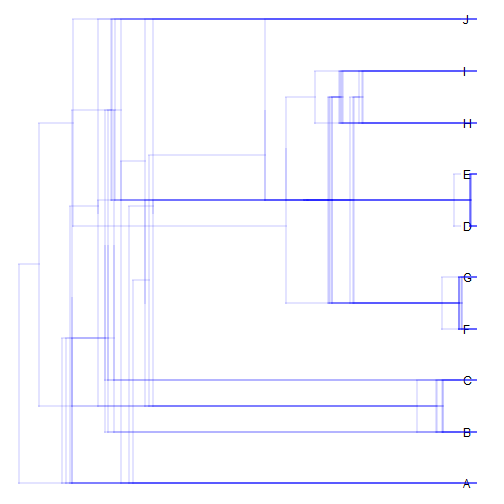Oops! That's no good.

Anyhow, I'm going to try & fix that as well as add some additional features to the function, and in so doing I intend to employ some of the tricks that I used in today's post.

Here is the re-written function:

``````## function to be used internally
rescaleTree<-function(tree,scale){
tree\$edge.length<-tree\$edge.length/max(nodeHeights(tree))*scale
tree
}
## densityTree function
densityTree<-function(trees,colors="blue",alpha=NULL,method="plotTree",
fix.depth=FALSE,use.edge.length=TRUE,compute.consensus=TRUE,
N<-length(trees)
if(any(sapply(trees,function(x) is.null(x\$edge.length))))
use.edge.length<-FALSE
if(!use.edge.length) trees<-lapply(trees,compute.brlen)
h<-sapply(trees,function(x) max(nodeHeights(x)))
if(fix.depth){
if(method=="plotTree"){
trees<-lapply(trees,rescaleTree,mean(h))
class(trees)<-"multiPhylo"
} else if(method=="plotSimmap"){
trees<-rescaleSimmap(trees,depth=mean(h))
print(class(trees))
print(class(trees[]))
}
h<-sapply(trees,function(x) max(nodeHeights(x)))
}
tips<-setNames(1:Ntip(trees[]),
if(compute.consensus) untangle(consensus(trees),
else trees[]\$tip.label)
if(is.null(alpha)) alpha<-max(c(1/N,0.01))
args<-list(...)
args\$direction<-"leftwards"
args\$tips<-tips
if(is.null(args\$nodes)) args\$nodes<-"inner"
if(is.null(args\$mar)) args\$mar<-if(show.axis) c(4.1,1.1,1.1,1.1) else rep(1.1,4)
if(is.null(args\$ftype)) args\$ftype<-"i"
plotTree(trees[[which(h==max(h))]],direction="leftwards",mar=args\$mar,
plot=FALSE)
args\$xlim<-get("last_plot.phylo",envir=.PlotPhyloEnv)\$x.lim[2:1]
if(method=="plotTree"){
args\$color<-make.transparent(colors,alpha)
for(i in 1:length(trees)){
args\$tree<-trees[[i]]
do.call(plotTree,args)
if(i==1){
if(show.axis) axis(1)
args\$ftype<-"off"
}
}
} else if(method=="plotSimmap"){
states<-sort(unique(as.vector(mapped.states(trees))))
print(states)
print(colors)
if(length(colors)!=length(states)){
colors<-setNames(c("grey",palette()[2:length(states)]),
states)
}
colors<-sapply(colors,make.transparent,alpha=alpha)
args\$colors<-colors
for(i in 1:length(trees)){
args\$tree<-trees[[i]]
do.call(plotSimmap,args)
if(i==1){
if(show.axis) axis(1)
args\$ftype<-"off"
}
}
}
nulo<-capture.output(rf<-multiRF(trees),type="message")
mds<-cmdscale(rf,k=1)[,1]
trees<-trees[order(mds)]
args\$ylim<-c(0,Ntip(trees[])+1)
plotTree(trees[[which(h==max(h))]],direction="leftwards",mar=args\$mar,
ylim=args\$ylim,plot=FALSE)
args\$xlim<-get("last_plot.phylo",envir=.PlotPhyloEnv)\$x.lim[2:1]
colors<-sapply(rainbow(n=length(trees)),make.transparent,alpha=alpha)
ftype<-args\$ftype
for(i in 1:length(trees)){
y.shift<-(i-median(1:length(trees)))/length(trees)/2
args\$tree<-trees[[i]]
args\$tips<-tips+y.shift
args\$color<-colors[i]
args\$ftype<-if(i==floor(median(1:length(trees)))) ftype else "off"
do.call(plotTree,args)
if(i==1){
if(show.axis) axis(1)
args\$ftype<-"off"
}
}
}
}
``````

We can try it out using various options. Note, to follow along you will have to update phytools from GitHub.

First, the basic method:

``````densityTree(trees)
``````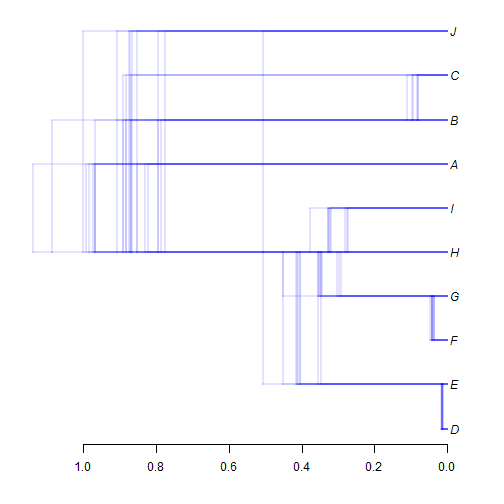or fix the total depth to be a constant value:

``````densityTree(trees,color="red",fix.depth=TRUE)
``````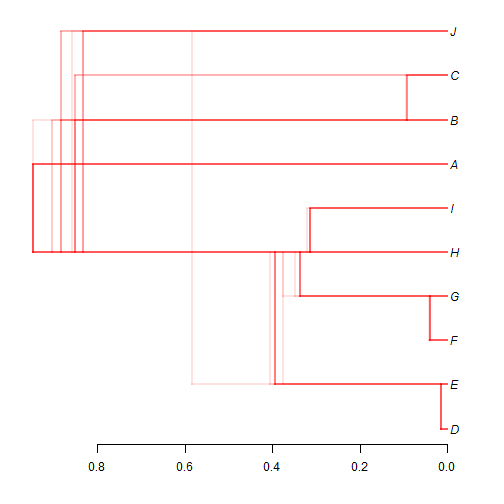We can also use a color gradient to be able to better distinguish the different topologies. I demonstrated this option today. I also added the feature that the method first sorts the trees (in a way) by computing the Robinson-Foulds distances between topologies & the uses MDS to sort the trees into one dimension. That means that plotted trees with similar gradient colors tend to have more similar topologies!

``````densityTree(trees,use.gradient=TRUE,fix.depth=TRUE,alpha=0.2)
``````
``````## Some trees are rooted. Unrooting all trees.
``````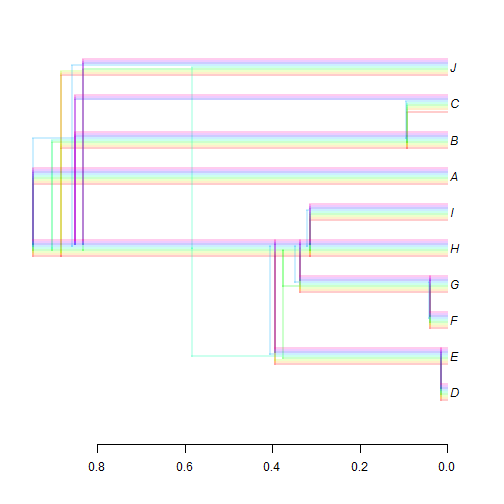This function also allows a mapped discrete character by changing the argument `method` to `plotSimmap`. Let's try it:

``````mtrees
``````
``````## 90 phylogenetic trees with mapped discrete characters
``````
``````densityTree(mtrees,colors=setNames(c("blue","red"),letters[1:2]),method="plotSimmap",
nodes="intermediate")
``````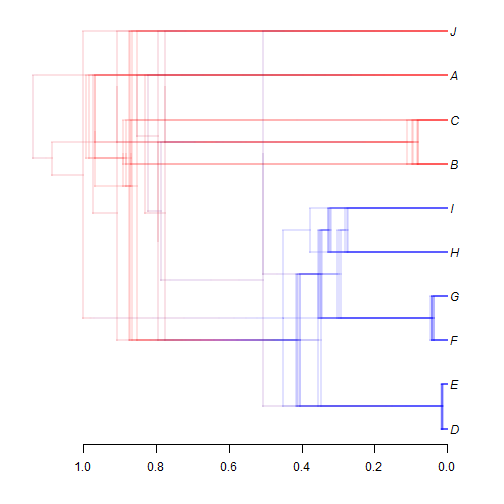Lastly, let's try it on a larger set of slightly bigger trees to see how it works:

``````tt
``````
``````## 99 phylogenetic trees
``````
``````densityTree(tt)
``````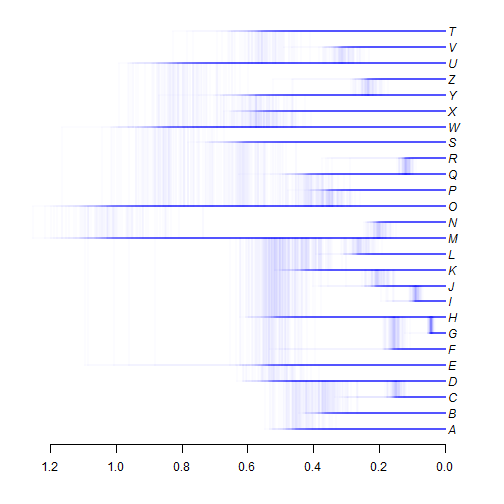``````densityTree(tt,use.gradient=TRUE,alpha=0.05,fix.depth=TRUE)
``````
``````## Some trees are rooted. Unrooting all trees.
``````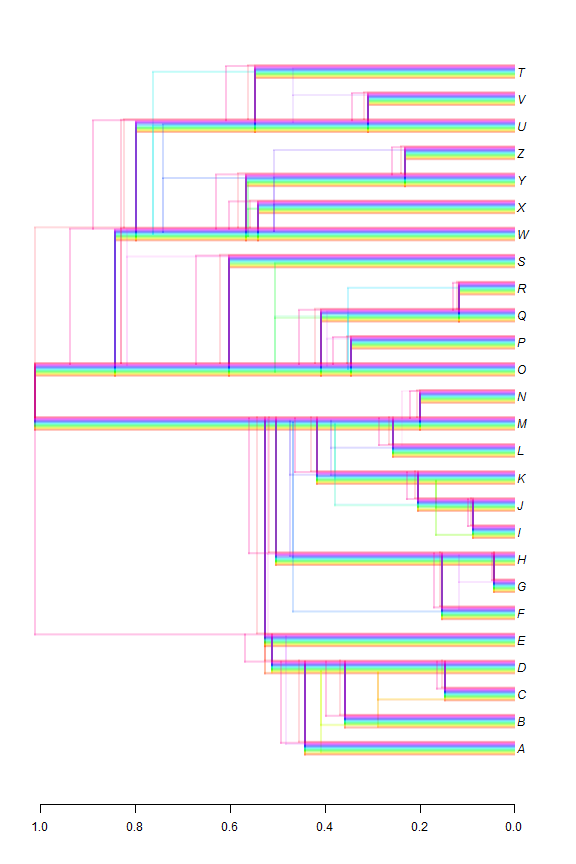Cool.

#### 1 comment:

1.How we can prun a densityTree like as species.trees

Note: due to the very large amount of spam, all comments are now automatically submitted for moderation.